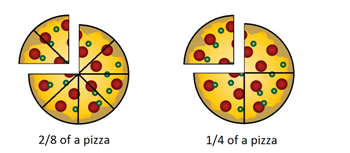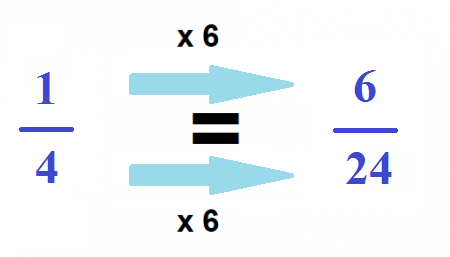# Equivalent Fractions: Lesson for Kids

Instructor: Rodney Davis

Rodney has 9 years of elementary teaching experience and a Master of Arts degree in Early Childhood Education.

In this lesson, we will discuss how fractions are equivalent. You will also learn how fractions can have the same value even though they may look totally different.

## What Are Equivalent Fractions?

Let's say that it's Friday night and your mom calls you to the table for dinner. To your delight, you're having pizza tonight! As you look around the table, you notice how the pizza, which comes in 8 slices total, has been divided among your family. There are 2 slices on your plate, and only 1 slice on your little brother's plate. Your mother has 2 slices, while your father has 3 slices.

Each of you have a fraction of the whole pizza. A fraction is a part of a whole. So, if you have 2 slices out of an 8-slice pizza, you would present it in the fraction form as 2/8.

So what are equivalent fractions? It might not be obvious at first, but 2/8 and 1/4 are equivalent fractions, which means that they represent the same amount of the whole--they are equal in value. That means that if your family's pizza were cut into only 4 pieces and you receive 1 piece, it would be the same amount of pizza as if you had received 2 of 8 pieces.## Finding Equivalent Fractions

There's a very simple trick to finding an equivalent for any fraction. The key is to multiply the numerator (the number on the top or left of the line of a fraction) and denominator (the number on the bottom or right of the line) of the fraction by the same whole number. The outcome will be an equivalent fraction. Let's look at an equivalent fraction for 1/4 found by multiplying it by 6:You can also find equivalent fractions by dividing the numerator and denominator of a fraction by the same number. For example, if we divide both sides of 6/10 by 2, we get 3/5. And so, 6/10 and 3/5 are equivalent fractions.

To unlock this lesson you must be a Study.com Member.

### Register to view this lesson

Are you a student or a teacher?

#### See for yourself why 30 million people use Study.com

##### Become a Study.com member and start learning now.
Back
What teachers are saying about Study.com

### Earning College Credit

Did you know… We have over 200 college courses that prepare you to earn credit by exam that is accepted by over 1,500 colleges and universities. You can test out of the first two years of college and save thousands off your degree. Anyone can earn credit-by-exam regardless of age or education level.